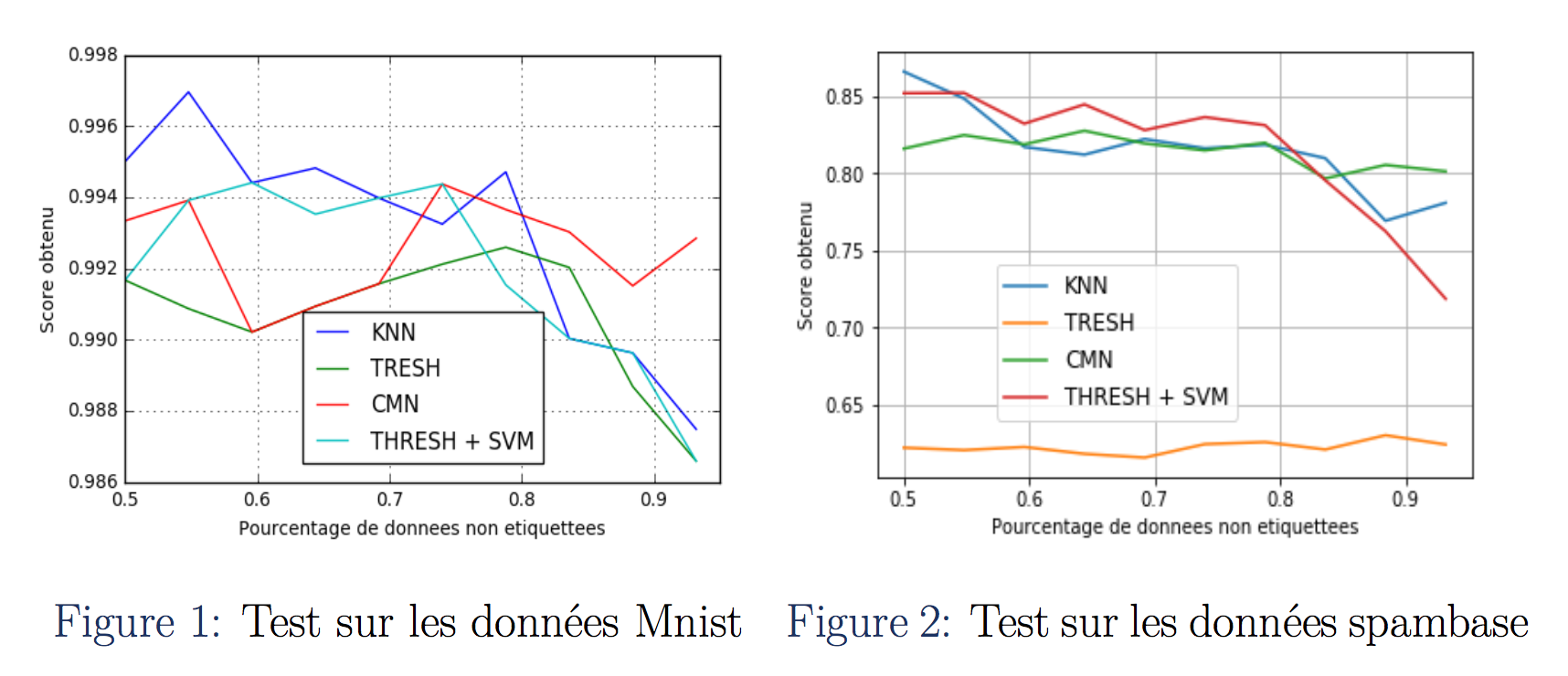# Semi-Supervised Learning With Harmonic Functions

Published:In many machine learning algorithms, we get predictions from labeled datas. Since data annotation is very expensive, the idea of using a small amount of datas to label other datas becomes quite hot. In this project, we intend to solve this problem using gaussian field and harmonic functions. We implemented and improved the algorithms proposed in the article Semi-Supervised Learning Using Gaussian Fields and Harmonic Functions.

## Requirement

• Python 2.7, Numpy, Scipy
• Jupyter notebook

## Algorithm

All the algorithms are situable for multi-class classification problems.

• Semi-supervised learning with a fixed threshold and a fixed weight matrix
• Semi-supervised learning in consideration of the proportion of each class with a fixed weight matrix
• Semi)supervised learning with an external classifier and a fixed weight matrix
• Learning the weight matrix

## Run

Please run the code in file: Projet.ipynb